# Shepherd

The shepherd has fewer than 500 sheep; where they can be up to 2, 3, 4, 5, 6 row is always 1 remain, and as can be increased up to 7 rows of the sheep, and it is not increased no ovine. How many sheep has a shepherd?

n =  301

### Step-by-step explanation:

a%b = a modulo b

n=7: n%2=1, n%3=1, n%4=3, n%5=2, n%6=1, n%7=0
n=14: n%2=0, n%3=2, n%4=2, n%5=4, n%6=2, n%7=0
n=21: n%2=1, n%3=0, n%4=1, n%5=1, n%6=3, n%7=0
n=28: n%2=0, n%3=1, n%4=0, n%5=3, n%6=4, n%7=0
n=35: n%2=1, n%3=2, n%4=3, n%5=0, n%6=5, n%7=0
n=42: n%2=0, n%3=0, n%4=2, n%5=2, n%6=0, n%7=0
n=49: n%2=1, n%3=1, n%4=1, n%5=4, n%6=1, n%7=0
n=56: n%2=0, n%3=2, n%4=0, n%5=1, n%6=2, n%7=0
n=63: n%2=1, n%3=0, n%4=3, n%5=3, n%6=3, n%7=0
n=70: n%2=0, n%3=1, n%4=2, n%5=0, n%6=4, n%7=0
n=77: n%2=1, n%3=2, n%4=1, n%5=2, n%6=5, n%7=0
n=84: n%2=0, n%3=0, n%4=0, n%5=4, n%6=0, n%7=0
n=91: n%2=1, n%3=1, n%4=3, n%5=1, n%6=1, n%7=0
n=98: n%2=0, n%3=2, n%4=2, n%5=3, n%6=2, n%7=0
n=105: n%2=1, n%3=0, n%4=1, n%5=0, n%6=3, n%7=0
n=112: n%2=0, n%3=1, n%4=0, n%5=2, n%6=4, n%7=0
n=119: n%2=1, n%3=2, n%4=3, n%5=4, n%6=5, n%7=0
n=126: n%2=0, n%3=0, n%4=2, n%5=1, n%6=0, n%7=0
n=133: n%2=1, n%3=1, n%4=1, n%5=3, n%6=1, n%7=0
n=140: n%2=0, n%3=2, n%4=0, n%5=0, n%6=2, n%7=0
n=147: n%2=1, n%3=0, n%4=3, n%5=2, n%6=3, n%7=0
n=154: n%2=0, n%3=1, n%4=2, n%5=4, n%6=4, n%7=0
n=161: n%2=1, n%3=2, n%4=1, n%5=1, n%6=5, n%7=0
n=168: n%2=0, n%3=0, n%4=0, n%5=3, n%6=0, n%7=0
n=175: n%2=1, n%3=1, n%4=3, n%5=0, n%6=1, n%7=0
n=182: n%2=0, n%3=2, n%4=2, n%5=2, n%6=2, n%7=0
n=189: n%2=1, n%3=0, n%4=1, n%5=4, n%6=3, n%7=0
n=196: n%2=0, n%3=1, n%4=0, n%5=1, n%6=4, n%7=0
n=203: n%2=1, n%3=2, n%4=3, n%5=3, n%6=5, n%7=0
n=210: n%2=0, n%3=0, n%4=2, n%5=0, n%6=0, n%7=0
n=217: n%2=1, n%3=1, n%4=1, n%5=2, n%6=1, n%7=0
n=224: n%2=0, n%3=2, n%4=0, n%5=4, n%6=2, n%7=0
n=231: n%2=1, n%3=0, n%4=3, n%5=1, n%6=3, n%7=0
n=238: n%2=0, n%3=1, n%4=2, n%5=3, n%6=4, n%7=0
n=245: n%2=1, n%3=2, n%4=1, n%5=0, n%6=5, n%7=0
n=252: n%2=0, n%3=0, n%4=0, n%5=2, n%6=0, n%7=0
n=259: n%2=1, n%3=1, n%4=3, n%5=4, n%6=1, n%7=0
n=266: n%2=0, n%3=2, n%4=2, n%5=1, n%6=2, n%7=0
n=273: n%2=1, n%3=0, n%4=1, n%5=3, n%6=3, n%7=0
n=280: n%2=0, n%3=1, n%4=0, n%5=0, n%6=4, n%7=0
n=287: n%2=1, n%3=2, n%4=3, n%5=2, n%6=5, n%7=0
n=294: n%2=0, n%3=0, n%4=2, n%5=4, n%6=0, n%7=0
n=301: n%2=1, n%3=1, n%4=1, n%5=1, n%6=1, n%7=0 <<<<<<=====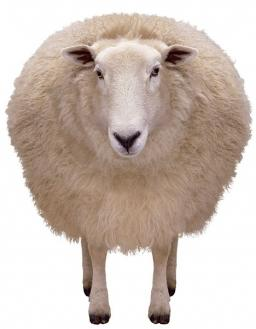Did you find an error or inaccuracy? Feel free to write us. Thank you!Tips to related online calculators
Do you want to calculate greatest common divisor two or more numbers?

## Related math problems and questions:

• Sheep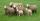Shepherd tending the sheep. Tourists asked him how much they have. The shepherd said, "there are fewer than 500. If I them lined up in 4-row 3 remain. If in 5-row 4 remain. If in 6-row 5 remain. But I can form 7-row." How many sheep have herdsman?
• Fewer than 500 sheep,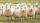There are fewer than 500 sheep, but if they stand in a double, triple, quadruple, five, and sixth-order, one sheep will remain. But they can stand in the seventh order. How many are sheep?
• Lcm = 22 + gcdThe least common multiple of two numbers is 22 more than their greatest common divisor. Find these numbers.
• TissuesThe store got three kinds of tissues - 132 children, 156 women and 204 men. Tissues each species were packed into boxes after the number of pieces the same for all three types (and greatest). Determine the number, if you know that every box has more than
• Bundle of candies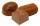In the store has 168 chocolates, caramel candies 224 and 196 hard candies. How many packages we can do and how many of candies will be in each package?
• 9.A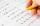9.A to attend more than 20 students but fewer than 40 students. A third of the pupils wrote a math test to mark 1, the sixth to mark 2, the ninth to mark 3. No one gets mark 4. How many students of class 9.A wrote a test to mark 5?
• PegsFrom two sticks 240 cm and 210 cm long, it is necessary to cut the longest possible pegs for flowers so that no residues remain. How many pegs will it be?
• Reminder and quotientThere are given the number C = 281, D = 201. Find the highest natural number S so that the C:S, D:S are with the remainder of 1,
• LCD 2The least common denominator of 2/5, 1/2, and 3/4
• Common divisorsFind all common divisors of numbers 30 and 45.
• Bricks pyramidHow many 50cm x 32cm x 30cm brick needed to built a 272m x 272m x 278m pyramid?
• Four poplarsFour poplars are growing along the way. The distances between them are 35 m, 14 m, and 91 m. At least how many poplars need to be dropped to create the same spacing between the trees? How many meters will it be?
• Gcd and lcm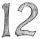Calculate the greatest common divisor and the least common multiple of numbers. a) 16 and 18 b) 24 and 22 c) 45 and 60 d) 36 and 30
• Shepherd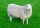Kuba makes a deal with a shepherd to take care of his sheep. Shepherd said to Kuba that he would receive twenty gold coins and one sheep after a year of service. But Kuba resigned just after the seventh month of service. But shepherd rewarded him and paid
• Diofant equationIn the set of integers (Z) solve the equation: 212x +316y =0 Write result with integer parameter t in Z (parameter t = ...-2,-1,0,1,2,3... if equation has infinitely many solutions)
• Florist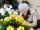Florist has 84 red and 48 white roses. How many same bouquets can he make from them if he must use all roses?
• School booksAt the beginning of the school year, the teacher distributed 480 workbooks and 220 textbooks. How many pupils could have the most in the classroom?